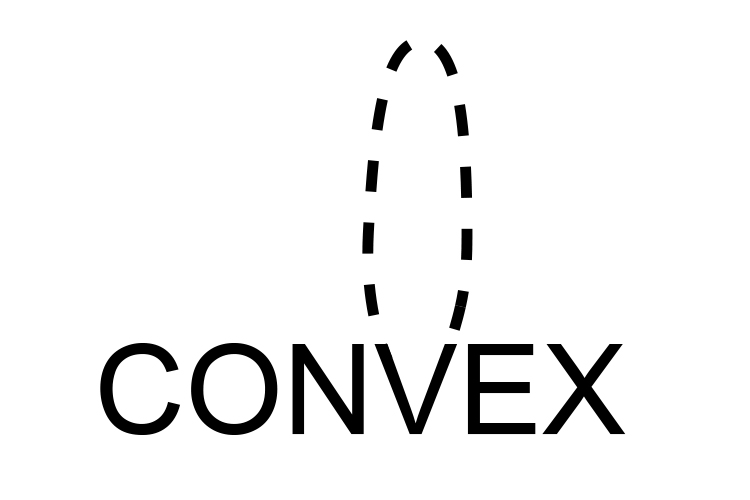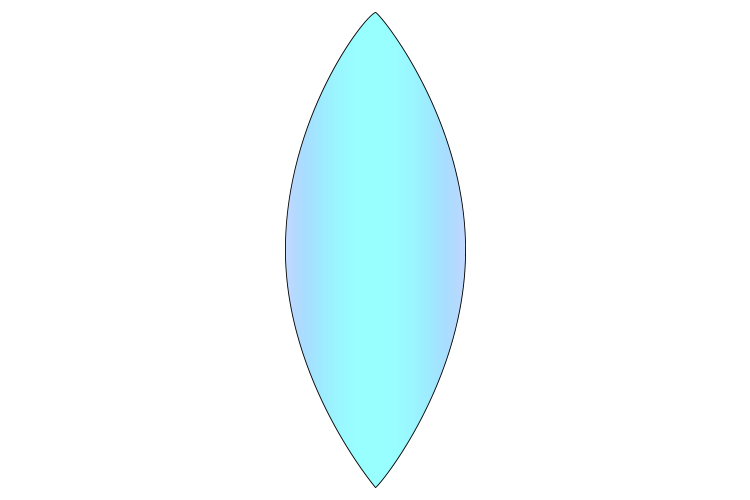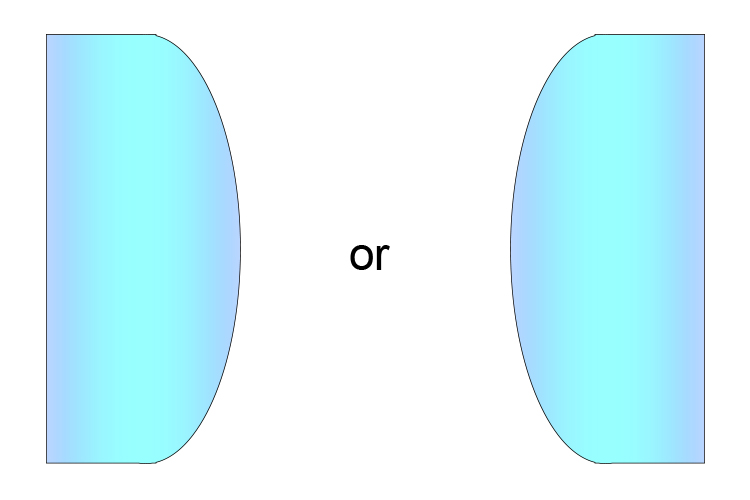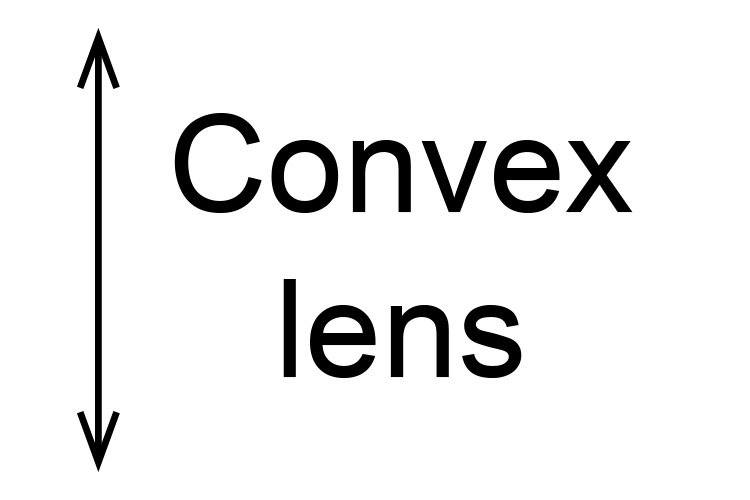# Convex lens

Look at the middle letter of convex and you can see it is the start of a convex surface.A CONVEX SURFACE CURVES OUTWARD

Convex surfaces curve outward and it tells you in the name.

A convex lens looks like the following:But because convex lenses are defined as being thinner at the edges and thicker in the middle, a convex lens only needs to have one surface that curves outwards: the other can be flat.A convex lens is a lens that possesses at least one surface that curves outwards.

NOTE: The above diagram shows convex lenses called plano convex lenses. An easy way to recall this is to remember that one surface is a plane (flat) and the other side looks like half an "O".

Plane "O" = Plano convex

Convex lenses are sometimes denoted by a symbol:This is drawn as a vertical line with outward facing arrows to indicate the shape of the lens is thicker in the middle.